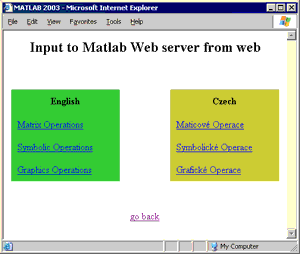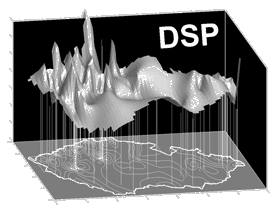Matlab Web Server
 The MATLAB® Web Server enables you to create MATLAB applications that use the capabilities of the World Wide Web to send data to MATLAB for computation and to display the results in a Web browser. The MATLAB Web Server depends upon TCP/IP networking for transmission of data between the client system and MATLAB. The required networking software and hardware must be installed on your system prior to using the MATLAB Web Server.
 Official demo of MATLAB Web Server Try out possibilities of MATLAB Web server in demo programs included in installation of that toolbox. MathWorks.com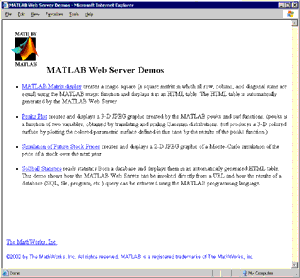Application of Autoregressive Models and Artificial Neural Networks in Time Series Prediction The focus real application of author's diploma thesis is on the mutual comparison of properties of different prediction methods, based on autoregressive modelling and linear, feed forward and recurrent neural network. All these models have been used in the adaptive and in the classical prediction approach. The algorithms of SVD and QRcp have been used to find the subset of the full autoregressive model. The same full set has been then used for three different types of neural networks. Many types of networks architectures with a wide range of modifications of the learning algorithms have been tested in the next stage. Suggested algorithms have been then applied for real data representing gas consumption in the Czech Republic with the focus on the winter part of the year. Spectral analysis of these signals with the sampling period of 2 hours and 1 day has been performed at first for original data and then for pre-processed signals. The main part of the work has been devoted to the discussion and comparison of all models mentioned above. Aleš Pavelka (May 2002)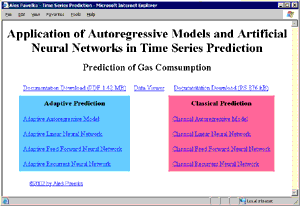Implementation of a MATLAB Web Server for Internet-based Processing of Biomedical Images The application is devoted to the presentation of possibilities of the MATLAB environment using the MATLAB Web Server. WWW pages and MATLAB programs have been created giving the opportunity to try simple programming only using the Internet browser. It is obvious that this approach to MATLAB has its own specific limitations and it does not replace MATLAB environment by itself. In this way it is very suitable for presentation of fundamental MATLAB structures and for courses devoted to studies of basic algorithms and main programming principles. Ing. Viktor Musoko (October 2003)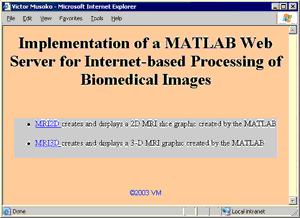Input to Matlab Web server from web Ing. Aleš Pavelka (October 2003)